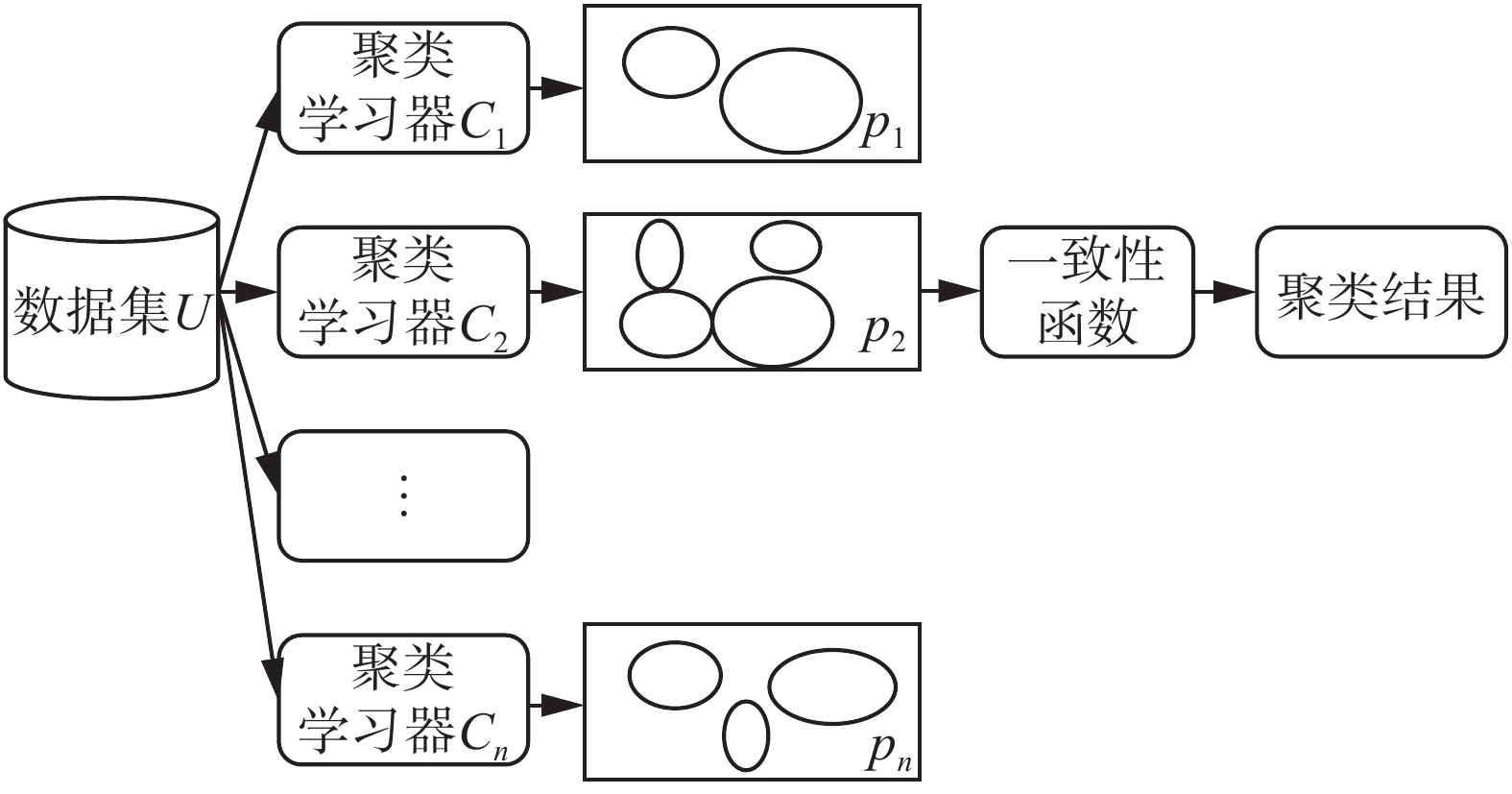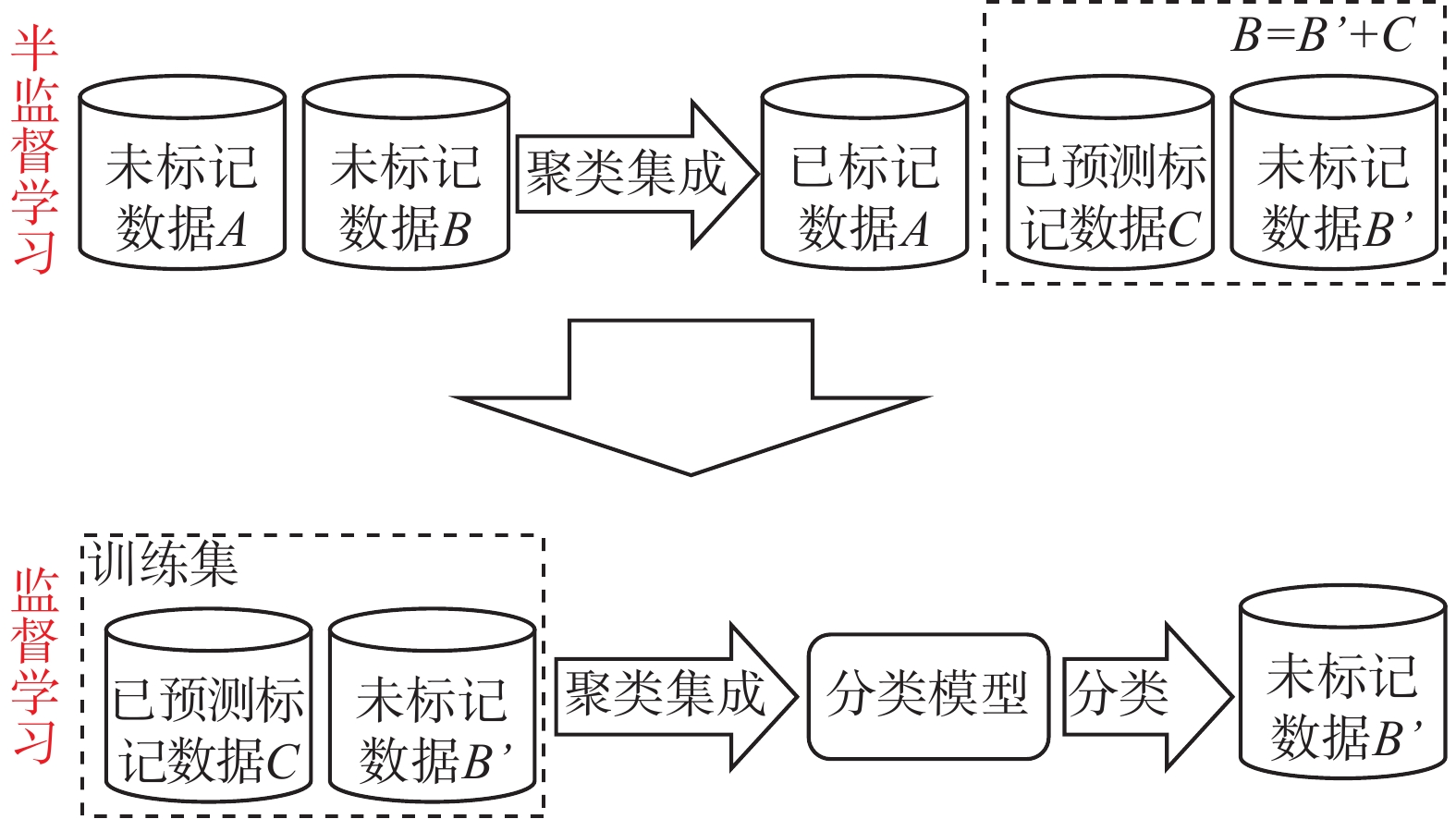﻿ SUCE：基于聚类集成的半监督二分类方法
«上一篇文章快速检索 高级检索

 智能系统学报2018, Vol. 13Issue (6): 974-980  DOI: 10.11992/tis.2017110270

### 引用本文MIN Fan, WANG Hongjie, LIU Fulun, et al. SUCE: semi-supervised binary classification based on clustering ensemble[J]. CAAI Transactions on Intelligent Systems, 2018, 13(6): 974-980. DOI: 10.11992/tis.201711027.### 文章历史

SUCE：基于聚类集成的半监督二分类方法

SUCE: semi-supervised binary classification based on clustering ensemble
MIN Fan, WANG Hongjie, LIU Fulun, WANG Xuan
School of Computer Science, Southwest Petroleum University, Chengdu 610500, China
Abstract: Semi-supervised learning and ensemble learning are important methods in the field of machine learning. Semi-supervised learning utilize unlabeled samples, while ensemble learning combines multiple weak learners to improve classification accuracy. This paper proposes a new method called Semi-sUpervised classification through Clustering and Ensemble learning (SUCE) for symbolic data. Under different parameter settings, a number of weak learners are generated using multiple clustering algorithms. Using existing class label information the weak learners are evaluated and selected. The test sets are pre-classified by weak learners ensemble. The samples with high confidence are moved to the training set, and the other samples are classified through the extended training set by using the basic algorithms such as ID3, Nave Bayes, kNN, C4.5, OneR, Logistic and so on. The experimental on the UCI datasets results show that SUCE can steadily improve the accuracy of most of the basic algorithms when there are fewer training samples.
Key words: ensemble learning    clustering    clustering ensemble    semi-supervised    binary classification

1 基本概念

 $S = \left( {U,C,d} \right)$ (1)Download: 图 1 聚类集成过程示意图 Fig. 1 The diagram of clustering ensemble

 ${\rm PC}\left( {{U_r}} \right) = \frac{1}{{\left| {{U_r}} \right|}}\mathop \sum \limits_{i = 1}^{\left| {{U_r}} \right|} \sigma \left( {t\left( {{x_i}} \right),d\left( {{x_i}} \right)} \right)$ (2)

 $\sigma \left( {a,b} \right) = \left\{ {\begin{array}{*{20}{c}}{1,\;\;\;\;\;\;a = b}\\{0,\;\;\;\;\;\;{\text{其他}}}\end{array}} \right.$ (3)

 $A\left( {{U_t}} \right) = \left\{ \begin{array}{l}{\bf normal}\left( {{U_t}} \right),\;\;\;\;{\rm PC}\left( {{U_r}} \right) > \theta \\{\bf covert}\left( {{U_t}} \right),\;\;\;\;{\rm PC}\left( {{U_r}} \right) < 1 - \theta \\{\rm reset}\left( {{U_t}} \right),\;\;\;\; 1 - \theta < {\rm PC}\left( {{U_r}} \right) < \theta \end{array} \right.$ (4)

1) 如果t(U)=[1 0 1 10], PC(Ur)=1>θ，所以 ${{d}}'\left( {{U_t}} \right) = {\bf normal}\left( {{U_t}} \right) = \left[ {1\;{\rm{}}0} \right]$

2) 如果t(U)=[0 1 0 0 1]，PC(Ur)=0<1−θ，所以 ${{d}}'\left( {{U_t}} \right) = {\bf convert}\left( {{U_t}} \right) = \left[ {1\;{\rm{}}0} \right]$

3) 如果t(U)=[0 0 0 0 1]，1-θ<PC(Ur)=0<1−θ，所以 ${{d}}'\left( {{U_t}} \right) = {\rm reset}\left( {{U_t}} \right) = \left[ { - 1\;{\rm{}} - 1} \right]$

 h\left( x \right) = \left\{ {\begin{aligned}&{d'\left( x \right),\;\;\;\displaystyle\mathop \sum \limits_{i = 1}^{\left| {\mathbb{C}} \right|} {d_i}'\left( x \right) = \left| C \right|\;{\rm{or}}\;\mathop \sum \limits_{i = 1}^{\left| {\mathbb{C}} \right|} {d_i}'\left( x \right) = 0}\\&{ - 1,\;\;\;\;\;\;\;{\text{其他}}}\end{aligned}} \right. (5)

${U_t} = \left\{ {{y_1},{y_2}} \right\}$ 的结果为

2 算法设计与分析

2.1 算法总体方案Download: 图 2 基于聚类集成的半监督分类示意图 Fig. 2 The diagram of semi-supervised classification based on clustering ensemble
2.2 算法描述

1) 通过getLabel(Ur)获取训练集Ur的标签 ${{{{L}}_{{U_r}}}}$ 。然后，利用remove(Ur)对Ur去标签得到Ur′；并将Ur′∪Ut得到无标签U

2) 通过多个基于EM、K-Means、FarthestFirst和HierarchicalClusterer等聚类算法的个体学习器对U进行全局聚类。根据已获取的 ${{{L}}_{U_r}}$ ，计算第i个聚类学习器 ${{{L}}_l}$ Ur上的聚类纯度PC(i)。如果PC(i)高于阈值θ ${{{L}}_l}$ 将继续参加集成学习，并将 ${{{L}}_l}$ 移入到学习器集合E中即E ${{{L}}_l}$

3) 对测试集的预测标签进行集成学习。通过h(x)一致性函数依次对测试集每个样本xUt的预测标签进行一致性处理。如果E中所有学习器对x的预测标签均一致，将预测标签d'(x)赋给x得到x'=(x, d'(x))。x'移入到训练集Ur∪{x'}，同时在测试集中将其删除Ut-{x}。

4) 对扩大规模后的Ur进行学习，再对缩减规模后的Ut进行分类 ${{{{L}}_{{U_t}}}}$ =classifier(Ur, Ut)得到Ut的类标签 ${{{{L}}_{{U_t}}}}$ ；然后，获取Ur的标签 ${{{{L}}_{{U_r}}}}$ =getLabel(Ur)。最终得到U类标签 ${{{{L}}_{{U}}}}$ =combine( ${{{{L}}_{{U_t}}}}$ , ${{{{L}}_{{U_r}}}}$ )。

SUCE：基于集成聚类的半监督分类算法

1）U=Ø，E=Ø；//初始化

2） ${{{{L}}_{{U_r}}}}$ =getLabel(Ur) //获取Ur类标签

3） $U_r' = {\rm remove}\left( {{U_r}} \right)$ ；//隐藏Ur类标签

4） $U = U_r' \cup {U_t}$

5） ${{{{L}}_1}} = {\rm KMeans}\left( U \right)$

6） ${{{{L}}_2}} = {\rm EM}\left( U \right)$

7） ${{{{L}}_3}} = {\rm FarthestFirst}\left( U \right)$

8） ${{{{L}}_4}} = {\rm HierarchicalCluster}\left( U \right)$

9）for (i=0; i<4;i++) do //筛选基学习器

10）　if (PC(i)>θ) then

11）E ${{{{L}}_{l}}}$

12）end if

13）end for

14）for (each xUt) do //标签一致性处理

15）if ( $h\left( x \right) = d'\left( x \right)$ ) then

16） ${{{{L}}_{{U_t}\left( x \right)}}} = d'\left( x \right)$

17）else then

18） ${{{{L}}_{{U_t}\left( x \right)}}} = -1$

19）end if

20）end for

21）for (each xUt) do //扩充训练集

22）if ( ${{{{L}}_{{U_t}\left( x \right)}}} ! = - 1$ ) then

23）x'=(x, d'(x))

24）Ur∪{x'};

25）Ut-{x};

26）end if

27）end for

28） ${{{{L}}_{{U_t}}}}$ =classifier(Ur, Ut)；//分类

29） ${{{{L}}_{{U_r}}}}$ =getLabel(Ur)；

30） ${{{{L}}_{{U}}}}\$ =combine( ${{{{L}}_{{U_t}}}}$ , ${{{{L}}_{{U_r}}}}$ )；

2.3 复杂度分析

1) 对数据集进行去标签化预处理。在隐藏Ur类标签之前，需先记录其真实类标签，如第2)行所示再隐藏Ur中的类标签，如第(3)行所示。至此，需要对Ur进行两次遍历，共执行2n次计算。接下来是合并去标签后的UrUt，构建无标签论域U。第1阶段，计算机将共执行3n+m次运算，故该阶段的时间复杂度为O(n+m)。

2) 分别通过基于K-Means、EM、FarthestFirst和HierarchicalClusterer基学习器对U进行全局聚类，如第5)~8)行所示。其时间复杂度分别为O(kt(n+m))、O(ct(n+m))、O(k(n+m))、O((n+m)2lg(n+m))，然后计算基学习器的聚类纯度，并对其进行筛选，共执行n×|E|次运算，如第9)~13)行所示。所以，第2阶段的时间复杂度为 $O\left( {\left( {n + m} \right)\left( {ct + \left( {n + m} \right)} \right.} \right.$ $\left. {\left. {\lg \left( {n + m} \right)} \right)} \right)$

3) 对Ut中的对象进行一致化处理。遍历Ut中对象，共执行m次处理，如第14)-20)行所示。然后将Ut中置信度高的对象移入到Ur，如第21)~27)行所示，共执行2m次计算，故时间复杂度为O(m)。

4) 对扩展后的Ur进行学习，并对Ut进行分类。该阶段的时间复杂度根据所采用的具体分类算法变化而变化。

3 实验及分析

3.1 实验设置表 1 数据集的描述 Tab.1 Description of the data set
3.2 实验结果与分析Download: 图 3 SUCE-ID3在不同数据集上的分类比较 Fig. 3 The diagram of comparison of SUCE-ID3 classification on different datasets表 2 SUCE与基础算法分类精度对比 Tab.2 Comparing the classification accuracy of SUCE and basic algorithms

SUCE更适用于ID3、C4.5、OneR等基础算法。例如，在Sonar数据上，SUCE-C4.5获得了高达14%的精度提升。然而，SUCE对Naive Bayes算法的改进不明显。

4 结束语

  MITCHELL T M. 机器学习[M]. 曾华军, 张银奎, 译. 北京: 机械工业出版社, 2003. (0)  ZHU Xiaojin. Semi-supervised learning literature survey[R]. Madison: University of Wisconsin, 2008: 63–77. (0)  张晨光, 张燕. 半监督学习[M]. 北京: 中国农业科学技术出版社, 2013. (0)  周志华. 机器学习[M]. 北京: 清华大学出版社, 2016. (0)  NIGAM K, MCCALLUM A K, THRUN S, et al. Text classification from labeled and unlabeled documents using EM[J]. Machine learning, 2000, 39(2/3): 103-134. DOI:10.1023/A:1007692713085 (0)  SONG Yangqiu, ZHANG Changshui, LEE J, et al. Semi-supervised discriminative classification with application to tumorous tissues segmentation of MR brain images[J]. Pattern analysis and applications, 2009, 12(2): 99-115. DOI:10.1007/s10044-008-0104-3 (0)  FENG Wei, XIE Lei, Zeng Jia, et al. Audio-visual human recognition using semi-supervised spectral learning and hidden Markov models[J]. Journal of visual languages and computing, 2009, 20(3): 188-195. DOI:10.1016/j.jvlc.2009.01.009 (0)  SHAHSHAHANI B M, LANDGREBE D A. The effect of unlabeled samples in reducing the small sample size problem and mitigating the Hughes phenomenon[J]. IEEE transactions on geoscience and remote sensing, 1994, 32(5): 1087-1095. DOI:10.1109/36.312897 (0)  梁吉业, 高嘉伟, 常瑜. 半监督学习研究进展[J]. 山西大学学报: 自然科学版, 2009, 32(4): 528-534. LIANG Jiye, GAO Jiawei, CHANG Yu. The research and advances on semi-supervised learning[J]. Journal of Shanxi university: natural science edition, 2009, 32(4): 528-534. (0)  MERZ C J, ST CLAIR D C, BOND W E. Semi-supervised adaptive resonance theory (SMART2)[C]//Proceedings of 1992 International Joint Conference on Neural Networks. Baltimore, USA, 1992: 851–856. (0)  VEGA-PONS S, RUIZ-SHULCLOPER J. A survey of clustering ensemble algorithms[J]. International journal of pattern recognition and artificial intelligence, 2011, 25(3): 337-372. DOI:10.1142/S0218001411008683 (0)  蔡毅, 朱秀芳, 孙章丽, 等. 半监督集成学习综述[J]. 计算机科学, 2017, 44(6A): 7-13. CAI Yi, ZHU Xiufang, SUN Zhangli, et al. Semi-supervised and ensemble learning: a review[J]. Computer science, 2017, 44(6A): 7-13. DOI:10.11896/j.issn.1002-137X.2017.6A.002 (0)  曾令伟, 伍振兴, 杜文才. 基于改进自监督学习群体智能(ISLCI)的高性能聚类算法[J]. 重庆邮电大学学报: 自然科学版, 2016, 28(1): 131-137. ZENG Lingwei, WU Zhenxing, DU Wencai. Improved Self supervised learning collection intelligence based high performance data clustering approach[J]. Journal of Chongqing university of posts and telecommunications: natural science edition, 2016, 28(1): 131-137. (0)  STREHL A, GHOSH J. Cluster ensembles—a knowledge reuse framework for combining partitionings[J]. Journal of machine learning research, 2002, 3: 583-617. (0)  FRED A L N, JAIN A K. Data clustering using evidence accumulation[C]//Proceedings of the 16th International Conference on Pattern Recognition. Quebec, Canada, 2002: 276–280. (0)  ZHOU Zhihua. Ensemble Methods: Foundations and Algorithms[M]. Boca Raton: Taylor and Francis Group, 2012: 135–156. (0)  ZHANG Minling, ZHOU Zhihua. Exploiting unlabeled data to enhance ensemble diversity[J]. Data mining and knowledge discovery, 2013, 26(1): 98-129. DOI:10.1007/s10618-011-0243-9 (0)  MIN Fan, HU Qinghua, ZHU W. Feature selection with test cost constraint[J]. International journal of approximate reasoning, 2014, 55(1): 167-179. DOI:10.1016/j.ijar.2013.04.003 (0)  GIONIS A, MANNILA H, TSAPARAS P. Clustering aggregation[M]//SAMMUT C, WEBB G I. Encyclopedia of Machine Learning. Boston: Springer, 2011. (0)  罗会兰, 孔繁胜, 李一啸. 聚类集成中的差异性度量研究[J]. 计算机学报, 2007, 30(8): 1315-1324. LUO Huilan, KONG Fansheng, LI Yixiao. An analysis of diversity measures in clustering ensembles[J]. Chinese journal of computers, 2007, 30(8): 1315-1324. DOI:10.3321/j.issn:0254-4164.2007.08.013 (0)  杨草原, 刘大有, 杨博, 等. 聚类集成方法研究[J]. 计算机科学, 2011, 38(2): 166-170. YANG Caoyuan, LIU Dayou, YANG Bo, et al. Research on cluster aggregation approaches[J]. Computer science, 2011, 38(2): 166-170. DOI:10.3969/j.issn.1002-137X.2011.02.038 (0)  杨玉梅. 基于信息熵改进的K-means动态聚类算法[J]. 重庆邮电大学学报: 自然科学版, 2016, 28(2): 254-259. YANG Yumei. Improved K-means dynamic clustering algorithm based on information entropy[J]. Journal of Chongqing university of posts and telecommunications: natural science edition, 2016, 28(2): 254-259. (0)  JAMSHIDIAN M, JENNRICH R I. Standard errors for EM estimation[J]. Journal of the royal statistical society. series B, 2000, 62(2): 257-270. DOI:10.1111/rssb.2000.62.issue-2 (0)  DEEPSHREE A V, YOGISH H K. Farthest first clustering in links reorganization[J]. International journal of web and semantic technology, 2014, 5(3): 17-24. DOI:10.5121/ijwest (0)  RASHEDI E, MIRZAEI A. A hierarchical clusterer ensemble method based on boosting theory[J]. Knowledge-based systems, 2013, 45: 83-93. DOI:10.1016/j.knosys.2013.02.009 (0)